## Gauss meets Fermat

A Mathematical Explorer notebook by Ed Pegg Jr.

In the margin of his copy of Bachet's edition of Diophantus, Fermat wrote:

It is impossible to separate a cube into two cubes, or a biquadrate into two biquadrates, or in general any power higher than the second into powers of like degree; I have discovered a truly remarkable proof which this margin is two small to contain.

In 1816, Gauss wrote:

I confess that Fermat's theorem as an isolated proposition has very little interest for me, because I could easily lay down a multitude of such propositions, which one could neither prove nor dispose of.

Fermat-Wiles Theorem: If n>2, there are no solutions in the positive integers to the equation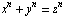.

One thing Gauss is famous for is the Gaussian Integers (the complex numbers a+b i such that a and b are integers). Are there any counterexamples to the Fermat-Wiles Theorem if one uses Gaussian Integers? This can be explored with The Mathematical Explorer. How about just looking at the cubic case, first?  One thing we will need is accurate cube roots of complex numbers. Every complex number has three different cube roots, and the one that is used by Round is not necessarily the best one.

````
`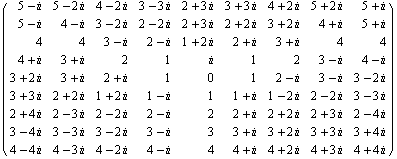`

We can use a mathematical trick to find the best cube root.

``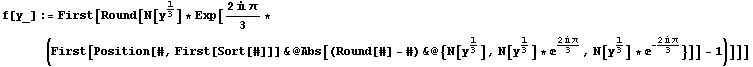``
``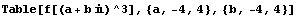``
`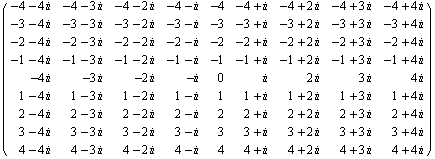`

Perfect.  I wonder what would happen if I connected each Gaussian Integer to the "closest" cube.  Hmm, this is kinda cool...

``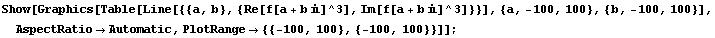``Now I'll be slightly sloppy, and add a lot of cubed complex numbers together.  First, I generate a table.  Next, I execute a Do loop, but with provisions to eliminate equivalent solutions.  I'm interested in how close we are getting to a counterexample.

``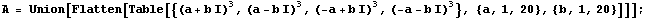``
````

It turns out that even among low numbers, it is easy to get very close to a counterexample.  And now it's late, so I'll stop here.

````
`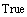`
````
``

Converted by Mathematica      September 11, 2001